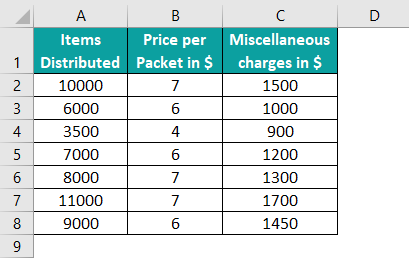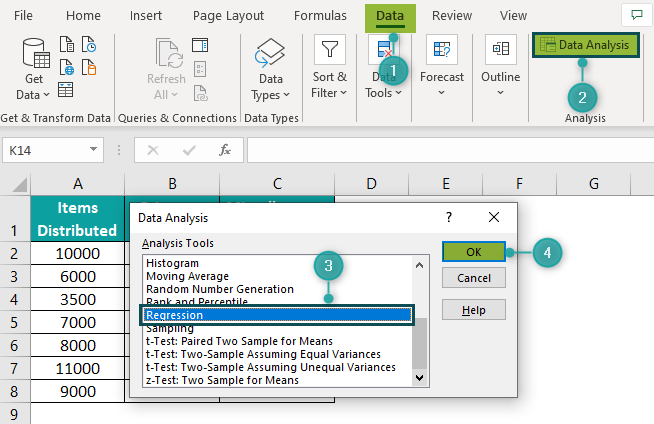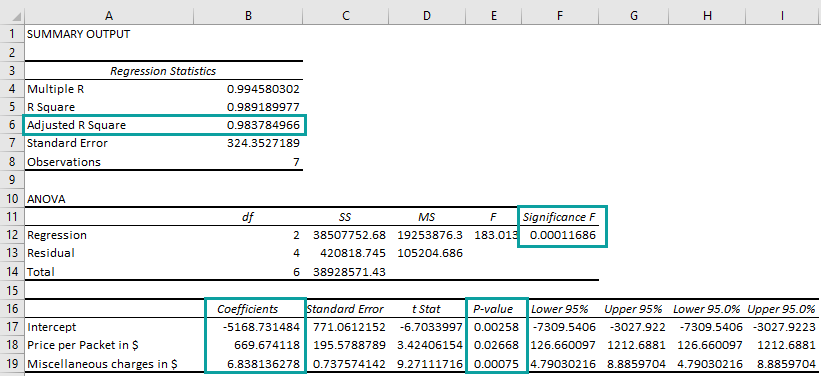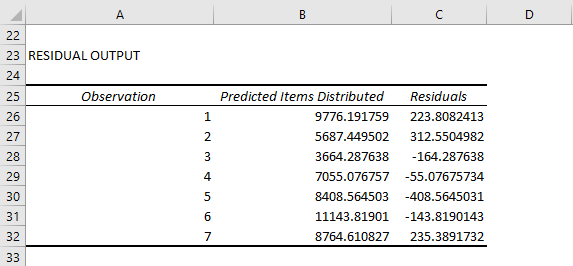# Regression Analysis In ExcelArticle byExcelMojo Team## What Is Regression In Excel?

Regression Analysis in Excel is a group of statistical methods. In simple terms, it evaluates the relationship between one dependent variable with one or more independent variables.

Also, it helps determine the strength of the estimated relationship and defines the future relationship between the variables. Thus, we can numerically assess how the fluctuations in the independent variables affect the dependent variable.

In addition, regression analysis is quite useful in finance.

For example, consider the table below showing income and savings in columns A and B.

Here, the Savings in \$ is the dependent, response, or outcome variable, whereas the Income in \$ is the independent, explanatory, or predictor variable.

Now, we need to estimate the dependent variable based on the independent variable.

So, if we plot a chart for regression analysis in Excel, we will get the result as shown in the image below:

In this case, the above chart is an example of linear regression analysis in Excel with a least square regression line. In addition, we can also see the regression equation in the chart area.

Clearly, we can infer from the chart that the weekly savings and income have a linear relationship.

Likewise, we can use regression analysis in excel to obtain the desired results.

##### Mathematical Representation

The mathematical representation is:

y = a + bx

where,

• y: Dependent variable
• a: Y-intercept
• b: Slope of the line
• x: Independent variable

Using this equation, we can predict the savings for different income values.

In the following sections, we will see how to determine the regression equation using the regression tool in Excel and then, perform a detailed regression analysis.

Likewise, if there are more independent variables apart from income, we can perform a multiple regression analysis in Excel. Also, it will show how savings change according to the fluctuations in the independent parameters.

###### Key Takeaways
• The regression analysis in Excel estimates the relationship between a dependent variable and independent variables using the least-squares regression method.
• We can install the Analysis ToolPak add-in, the regression tool, to perform the regression analysis in our worksheet.
• The regression tool helps us determine R Square, Significance F, and Coefficients. They are the most critical parameters required in a regression analysis.
• We can create a regression graph using the Scatter charts option. Alternatively, we can use regression formulas such as LINEST(), INTERCEPT(), SLOPE(), and CORREL() to perform the analysis.

### How To Run Regression Tool In Excel

We can perform a regression analysis in Excel using Analysis ToolPak. Even though it is an add-in, we need to install it in Excel to run the regression tool. Using the below steps, we can install and run the regression tool in Excel.

Step 1: First, choose the File tab.

Then, click on Options.

Step 2: Excel Options window pops up. Next, select Add-ins option from the menu.

Step 3: Now, we should ensure that the Excel Add-ins option is selected in the Manage box. Next, choose Analysis ToolPak in the Inactive Application Add-ins list and then, click Go.

Step 4: The Add-ins window pops up. Here, choose the Analysis ToolPak box and click OK.

Step 5: Then, go to the Data tab.

Clearly, we can see the Data Analysis option under the Analysis group.

Step 6: Now, we can use the Data Analysis option to run the regression tool in Excel.

Click on Data Analysis.

Step 7: The Data Analysis window pops up. Now, scroll down the Analysis Tools list; choose Regression, and click OK.

The Regression window appears. Here, we should enter the required parameters to obtain the regression analysis in Excel output.

The following sections help us understand how to enter the values and interpret the regression analysis output.

### How To Use Regression Tool In Excel?

Let us see how to use the tool to perform regression analysis in Excel. Remember, the program uses the least-squares regression technique for linear regression.

After we install the Analysis ToolPak, we need to follow the below steps:

• First, add the required table to the worksheet.
• Next, choose Data 🡪 Data Analysis from the ribbon.
• Select Regression from the Data Analysis window and click OK.
• Next, enter the cell ranges for dependent (Input Y Range) and independent (Input X Range) variables in the Regression dialog box.
• Then, click OK to view and analyze the result.

Let us look at an example to understand simple regression analysis in Excel using the regression tool.

The below table shows the demand and price details of a product recorded during the years 2010-22. Now, we need to use the following steps to understand simple regression analysis in Excel using the regression tool.

In the table,

• Column A shows the years
• Column B displays the demand for the product
• Column C shows the rate per carton

The steps to perform the regression analysis in Excel using the Analysis ToolPak are:

Step 1: To begin with, go to Data and choose Data Analysis from the Analysis group.

Step 2: Next, the Data Analysis window pops up. In this window, select Regression and click OK.

Step 3: Then, the Regression window appears. We must enter the required parameters to perform a simple regression analysis in Excel.

• First, we must choose the dependent and independent variable ranges for Input Y Range and Input X Range, respectively. For our table, the respective cell ranges are C1:C14 and B1:B14, as the changes in the price per carton depend on the fluctuations of the product’s demand.
• Also, choose the options Labels, New Worksheet Ply, and Residuals. They enable us to see the column labels in the output, which will help us understand the result. Also, it creates the residual information in a new worksheet.

Step 4: Click OK.

As soon as we click OK, we will be able to see the below output in a new worksheet. Also, it helps us conduct regression analysis in Excel.

The interpretation of the regression analysis output is as follows:

Summary Output

Multiple R

It denotes the Correlation Coefficient. In addition, the absolute value indicates how strong the linear relationship is between the two variables. The Multiple R value varies between -1 and 1, where -1 infers that the relationship is negative and 1 indicates that it is positive. 0 denotes that there is no relationship.

In our example, the value is 0.92, so the Rate Per Carton and Product Demand relationship is positive.

R Square

Referred to as the Coefficient of Determination, R Square is the summation of all squared deviations of the data points from the mean.

It shows the percentage of values that fits in the regression analysis model. A value of 0.95 (95%) or more is an excellent fit.

For our table, it is 0.86. So, it means that the value is acceptable.

The Adjusted R Square is the adjustment made to the R Square value considering the independent variable count. We will have to use it instead of R Square when performing a multiple regression analysis in Excel.

Standard Error

It shows whether the regression analysis and the corresponding equations are precise. Also, the Standard Error is the absolute value indicating how far the data points fall from the regression line on average. A smaller value denotes a more precise regression equation.

Observations

It is the total number of data points in the model.

ANOVA

It stands for Analysis of Variance. It shows the various components, the sum of squares, which explains the variability levels within the regression model.

Generally, we will not refer to this section for simple regression analysis. However, the parameter Significance F shows the statistical significance of our result. If it is more than 0.05, the suggestion is to perform regression analysis with another independent variable.

In our example, the value is lesser than 0.05, so we do not have to change the independent variable.

Coefficients

It is the most important section that helps develop the equation for linear regression analysis in Excel.

For our table, the equation will be:

y = Intercept + Product Demand [Number of Cartons] Coefficient * x

We can now substitute the variable x with a specific number of cartons as Product Demand and obtain the value of y, the associated Rate Per Carton.

Residuals

The calculated y value is an estimate and may differ from the actual number. The reason is that the independent variables cannot accurately predict the response variable.

The Residuals table shows the difference between the actual and estimated Rate per Carton values.

### Examples

Let us look at the following examples to understand regression analysis in Excel.

#### Example 1

Let us learn how to perform multiple regression analysis using regression tool in Excel.

The table below shows the number of straw packets sold, along with the rate per packet, and the associated marketing costs in columns A, B, and C, respectively.

The Straw Packets Sold value is the dependent variable, and the independent variables are Rate per Packet and Marketing Costs. So, we need to perform regression analysis in excel for this data.

The following steps help us determine the relationship between the dependent and predictor variables using regression analysis in Excel.

Step 1: First, select Data and choose Data Analysis from the Analysis group.

Step 2: Then, the window named Data Analysis pops up. Choose Regression and click OK.

Step 3: Next, the Regression window pops up.

Here, enter the cell ranges for the dependent and independent variables (Input Y Range and Input X Range).

Also, select the Labels, New Worksheet Ply, and Residuals options.

Click OK to view the output for multiple regression analysis.

Now, we will be able to see the below output in a new worksheet.

Please Note: The Adjusted R Square value is 0.9824. Therefore, it indicates that our model has an accuracy of 98.24%. So, it is a good fit.

The Significance F (0.0001363) and P-value (0.00367, 0.00575, 0.00221) are less than 0.05. Therefore, it implies that regression analysis with the two independent variables is ok, and our data is significant.

Also, as it is a multiple regression analysis, the equation for regression analysis in Excel in this example will be:

y = a + b0 * x0 + b1 * x1

Since there are two independent variables, we can see x0 and x1 with their respective slopes b0 and b1 in the equation.

Thus, the regression equation for our table is:

y = Intercept + Rate per Packet in \$ Coefficient * x0 + Marketing Costs in \$ Coefficient * x1

where,

• y: Straw Packets Sold
• Intercept: -3813.439 [Refer to Coefficients in the first image of Step 4]
• x0: Rate per Packet in \$
• x1: Marketing Costs in \$
• Rate per Packet in \$ Coefficient: 980.931 [Refer to Coefficients in the first image of Step 4]
• Marketing Costs in \$ Coefficient: 4.793 [Refer to Coefficients in the first image of Step 4]

We can predict y by substituting the x0 and x1, and by referring to the Residuals table, we can determine the deviation of the actual values from the predicted values.

#### Example 2

Let us learn how to derive residual plots using regression analysis in Excel.

Consider the below table with the total marks scored by students and the number of hours they spent studying for each exam in columns A and B.

Now, we need to analyze the relationship between the hours studied (predictor variable) and total scores (response variable) secured using regression analysis in Excel.

The steps used to analyze the relationship using regression analysis in Excel are as follows:

Step 1: First, click on the Data tab and choose Data Analysis from the Analysis group.

Step 2: Then, the Data Analysis window pops up.

Choose Regression and click OK.

Step 3: Next, the Regression window appears.

Step 4: Now, enter the cell ranges for the dependent and independent variables.

Also, check the Labels, New Worksheet Ply, Residuals, and Residual Plots options.

Then, click OK to view the residual plot and the regression analysis output.

Clearly, we can see that the residuals are scattered closer to zero throughout the fitted values’ stretch. So, it indicates that the model’s estimations are acceptable on average.

### How To Create Regression Graph?

We can perform regression analysis in Excel by creating a regression graph.

For example, consider the table below showing the number of flu cases and the available Tamiflu capsules’ stock in columns A and B, respectively.

Now, we can create the regression graph for analyzing the relationship between the two variables, Tamiflu Capsules [Number of Packets] and Flu Cases using regression analysis in Excel.

The steps used to create regression graph are as follows:

Step 1: First, we need to select both the columns along with the headers.

Step 2: Next, choose the Insert tab; Then, click on the Scatter Chart option from the Charts group.

Step 3: Excel has a lot of inbuilt scatter chart types. In this example, let us select the first chart type.

Then, the scatter plot will immediately appear on the worksheet.

Step 4: Now, right-click on one of the data points in the graph and select Add Trendline in the context menu to show the least-squares regression line.

Step 5: The trendlines appear in the plot.

Meanwhile, we can see the Format Trendline window on the right side of the worksheet. Choose Linear from the Trendline Options tab.

Now, scroll down and check the Display Equation on chart box.

Please Note: The regression equation we see in the chart area will be the same as the results obtained using the regression tool.

So now, we can perform the regression analysis in Excel using the graph.

Step 6: Also, we can make the regression graph more presentable by making appropriate changes in the Fill & Line tab.

For example, let us change the regression line color to Light Green and the Dash Type to Solid Line.

Please Note: To move the equation to another location in the chart area, we should keep the Format Trendline window open. Only then we can move it.

Step 7: Now, click on any scatter points to change the format. Immediately, the Format Trendline window changes to Format Data Series window.

Next, click on the Marker tab to change colors. In this example, let us change the color to Dark Blue.

Step 8: Now, click on the chart area. The Chart Elements window pops up. Select Axis Titles.

Then, name the X and Y axes and give an appropriate chart title. Now, our regression chart will appear as shown below:

### Regression Using Excel Formulas

Also, we can perform the regression analysis in Excel using statistical functions.

The regression equation requires the Y-intercept (a) and regression line slope (b). So, we need to determine the Coefficient Correlation (Multiple R).

Let us verify the determined regression equation with the Excel formulas for the same example where column A shows the number of flu cases and the available Tamiflu capsules’ stock in columns A and B, respectively.

LINEST()

The function utilizes the least-squares regression method for calculating the relationship between the concerned variables. Therefore, it returns an array describing the regression line.

So, let us choose two consecutive cells, say E2:F2, as the output will be an array.

Next, type the LINEST() formula and press Ctrl + Shift + Enter.

Please Note: We should select only the data sets and not the headers. Or else, we will get #VALUE! error.

After we press Ctrl + Shift + Enter, the output will be:

INTERCEPT()

The formula to determine the Y-intercept (a) is =INTERCEPT(B2:B11,A2:A11)

SLOPE()

The formula to determine the slope (b) is =SLOPE(B2:B11,A2:A11)

CORREL()

The formula to determine the Correlation Coefficient (Multiple R) is

=CORREL(B2:B11,A2:A11)

When we apply the above formulas, the output will be:

We get the same values for the Y-intercept and Slope. So, we obtain the same regression equation irrespective of the method used, i.e., using regression graph or formulas for regression analysis in Excel.

### Important Things To Note

• The regression analysis in Excel enables us to predict how a dependent variable will vary according to the fluctuations in the independent variables.
• To perform regression analysis correctly, first, we should identify and use the required dependent and independent variables.
• Next, we should check Adjusted R Square instead of R Square in the regression tool output when performing multiple linear regression analysis.
• If the parameter Significance F in our regression tool output is more than 05, then, we need to perform the regression analysis using different independent variables.

What is the slope in regression analysis in Excel?

The slope in regression analysis in Excel is the ratio of the vertical and horizontal distance between any two data points on the regression line. Also. it denotes the rate of change throughout the regression line.

How to do regression analysis in Excel with multiple variables?

We can do regression analysis in Excel with multiple variables.

First, install the Analysis ToolPak add-in in Excel. [Refer to the How to Run Regression Tool in Excel section]

For instance, let us perform the regression analysis for the below table:Here, the dependent variable is Items Distributed (the data in column A), and the multiple independent variables are the values in columns B and C, i.e., Price per Packet in \$ and Miscellaneous Charges in \$.

Step 1: To begin with, choose Data > Data Analysis from the Analysis group.

Next, the Data Analysis window pops up. Select Regression and then, click OK.Step 2: Then, the Regression window appears. Here, enter the cell range for the dependent variable in Input Y Range.

Also, provide the entire cell range, including all the independent variables, in the Input X Range.

Remember, the cell ranges should also include the column headers as it will help us interpret the regression analysis output better.

In addition, choose the options Labels, New Worksheet Ply, and Residuals.Step 3: Click OK to view the result.

Clearly, we can get a coefficient for each independent variable and the Intercept. Thus, we can build the regression equation for estimating the relationship between one response and multiple predictor variables.Interpretation: The Adjusted R Square value is 0.98, making the estimation good. The Significance F and P-value are below 0.05, so the data is significant. And the regression equation is:

y = -5168.731 + 669.674 * x0 + 6.838 * x1

where,

y: Items Distributed
x0 and x1: Price per Packet in \$ and Miscellaneous Charges in \$

What does the regression analysis in Excel tell you?

The regression analysis in Excel tells us how a dependent variable behaves according to the fluctuations in one or more independent variables and their future relationship.

Where is regression analysis in Excel?

The regression analysis in Excel is in the Data Analysis option in the Data tab. With just a few clicks, we can install the Analysis ToolPak add-in to enable the Data Analysis option.